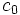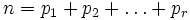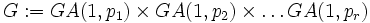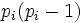# Orbital maximin is bounded below by constant fraction of number of ordered pairs of distinct elements for solvable groups

## Statement

Let$S$ be a set of size$n$, with$n \ge 2$. Consider the orbital maximin problem for the action of solvable groups on$S$, i.e., we want to find the maximum possible value of the smallest orbital size under a solvable group action on$S$. The total number of ordered pairs of distinct elements is$n(n - 1)$. The claim is that the following equivalent statements are true:

1. There is a constant$c$ such that the orbital maximin is$\ge n(n-1)/c$ for all$n$.
2. There is a constant$c_0$ such that, for all sufficiently large$n$, the orbital maximin is$\ge n(n-1)/c_0$.

## Proof

We prove (2).

### The general construction: additive partitioning

Consider an expression for$n$ as a sum of primes:$n = p_1 + p_2 + \dots + p_r$

Consider now the group:$G := GA(1,p_1) \times GA(1,p_2) \times \dots GA(1,p_r)$

where$GA(1,p_i)$ is the general affine group (also called the affine general linear group) over the prime field with$p_i$ elements. In other words, it is the semidirect product of the additive group of the field and the multiplicative group of the field. Note that each$GA(1,p_i)$ is a metabelian group, hence so is$G$.

We consider now the following action of$G$ on a set$S$ of size$n$. First, divide$S$ into subsets$T_i$ of size$p_i$ each. Identify each$T_i$ with the underlying set of the field of$p_i$ elements. Now, the action of an element of$G$ on the piece$T_i$ is the action of its$i^{th}$ coordinate viewed as an element of the affine group acting on the field.

The orbits of elements are the sets$T_i$, and there are two kinds of orbitals:

• The orbitals where both elements are in the same$T_i$: These have size$p_i(p_i - 1)$ because the action of the general affine group is a doubly transitive group action.
• The orbitals where the two elements are in different$T_i$s, say$T_i$ and$T_j$: These have size$p_ip_j$.

The minimum of all these turns out to be$p(p - 1)$ where$p$ is the minimum of the$p_i$s. Therefore, if we can guarantee a partition into primes where each of the primes is$\le n/K$ for some constant$K$, we will be done.

### Result from additive number theory

The result now follows from Haselgrove's strengthening of Vinogradov's theorem, which states that any odd integer$n$ can be divided into three primes that are$(n/3) + o(n)$, and an easy corollary which shows that any even integer$n$ can be divided into three primes that are$n/4 + o(n)$. This would show that any constant \$c_0 > 16[/itex] will work. Also, we see that our choice of$G$ has polycyclic breadth at most eight.

If strong versions of Goldbach's conjecture are true, we can push it down to any constant$c_0 > 4$.